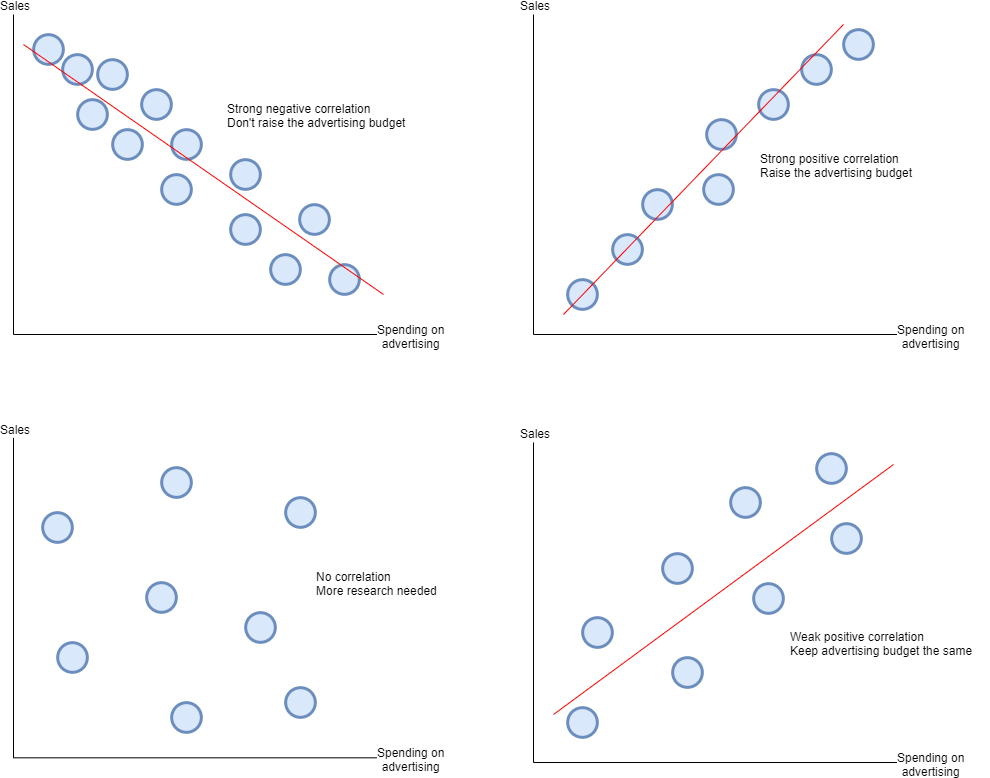Posted on

# 3.3.1 Quantitative sales forecasting

Components:

• Trend – Correlations.
• Cyclical – Due to economic factors.
• Seasonal – Changes in sales due to seasons.
• Irregular – Changes in sales due to irregular events e.g. hurricane.

A) Calculation of time-series analysis:

3 point moving averages

Variation = difference between actual sales and 3pma

Cyclical variation = add up the variation for each cycle point e.g. all cycle point 1’s then divide by the number of cycle points e.g. 3

Forecasting = The 3pma of the last cycle point that corresponds to the one that is being predicted e.g. cycle point 2 takeaway the cyclical variation of cycle point 2

4 point moving average

4 period moving total = Add up the top 4 cycle points of sales then move up one each time

4pma = 4pmt/number of cycle points (4)

Centred 4 period moving average = Add up the top four 4pma then divide by the cycle points (4) move down then continue doing this. Put the figure in-between the 4 4pma

Cyclical variation = difference between sales and the centred 4 period moving average

Cycle point average cyclical variations =  Add up the variation for each cycle point e.g. all cycle point 1’s then divide by the number of cycle points with variation figures available to them e.g. 2

Forecasting

The centred 4 period moving average takeaway the cycle point average cyclical variation that corresponds to that cycle point e.g. cycle point 2 takeaway the cyclical variation of cycle point 2.

B) Interpretation of scatter graphs and line of best fitC) Limitations of quantitative sales forecasting techniques

Past performance is no guarantee of the future – Changes in the market or fashions and trends may cause a change in sales differing from past sales.

Other factors can affect future predictions (PESTLE)

Relies on past data – This may not always a good indication of what may happen in the future.

Time consuming – Complex to make.